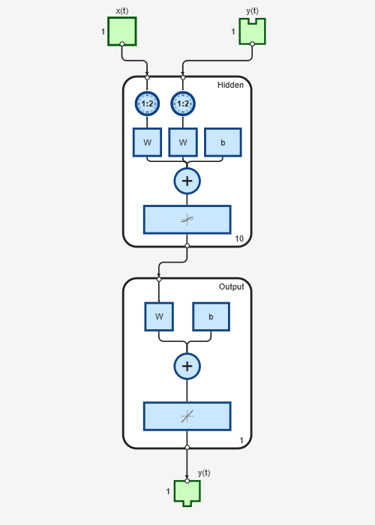# narxnet

Nonlinear autoregressive neural network with external input

## Syntax

```narxnet(inputDelays,feedbackDelays,hiddenSizes,feedbackMode,trainFcn) ```

## Description

NARX (Nonlinear autoregressive with external input) networks can learn to predict one time series given past values of the same time series, the feedback input, and another time series, called the external or exogenous time series.

`narxnet(inputDelays,feedbackDelays,hiddenSizes,feedbackMode,trainFcn)` takes these arguments,

 `inputDelays` Row vector of increasing 0 or positive delays (default = 1:2) `feedbackDelays` Row vector of increasing 0 or positive delays (default = 1:2) `hiddenSizes` Row vector of one or more hidden layer sizes (default = 10) `feedbackMode` One of `'open'`, `'closed'`, or `'none'` (default is `'open'`) `trainFcn` Training function (default is `'trainlm'`)

and returns a NARX neural network.

## Examples

collapse all

Partition the training data. Use `Xnew` to do prediction in closed loop mode later.

```[X,T] = simpleseries_dataset; Xnew = X(81:100); X = X(1:80); T = T(1:80); ```

Train a network, and simulate it on the first 80 observations

```net = narxnet(1:2,1:2,10); [Xs,Xi,Ai,Ts] = preparets(net,X,{},T); net = train(net,Xs,Ts,Xi,Ai); view(net) ```Calculate the network performance.

```[Y,Xf,Af] = net(Xs,Xi,Ai); perf = perform(net,Ts,Y) ```
```perf = 0.0153 ```

Run the prediction for 20 time steps ahead in closed loop mode.

```[netc,Xic,Aic] = closeloop(net,Xf,Af); view(netc) ``````y2 = netc(Xnew,Xic,Aic) ```
```y2 = 1x20 cell array Columns 1 through 5 {[-0.0156]} {[0.1133]} {[-0.1472]} {[-0.0706]} {[0.0355]} Columns 6 through 10 {[-0.2829]} {[0.2047]} {[-0.3809]} {[-0.2836]} {[0.1886]} Columns 11 through 15 {[-0.1813]} {[0.1373]} {[0.2189]} {[0.3122]} {[0.2346]} Columns 16 through 20 {[-0.0156]} {[0.0724]} {[0.3395]} {[0.1940]} {[0.0757]} ```